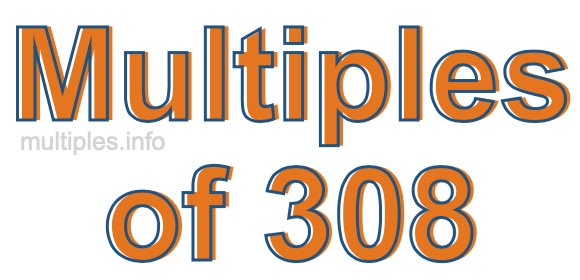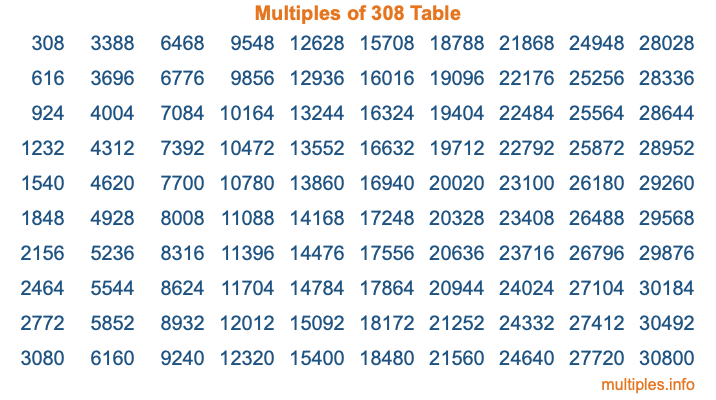Multiples of 308Welcome to the Multiples of 308 page. Here we will first teach you everything you will ever need to know about the multiples of 308, and then give you a study guide summary of everything we taught you to make sure you remember it all. Use this page to look up facts and learn information about the multiples of 308. This page will make you a multiples of three hundred eight expert!

Definition of Multiples of 308
Multiples of 308 are all the numbers that when divided by 308 equal an integer. Each of the multiples of 308 are called a multiple. A multiple of 308 is created by multiplying 308 by an integer.

Therefore, to create a list of multiples of 308, you start with 1 multiplied by 308, then 2 multiplied by 308, then 3 multiplied by 308, and so on for as long as you want. Thus, the list of the first five multiples of 308 is 308, 616, 924, 1232, and 1540. To see a larger list of multiples of 308, see the printable image of Multiples of 308 further down on this page. We also have a category where you can choose any nth multiple of 308.

Multiples of 308 Checker
The Multiples of 308 Checker below checks to see if any number of your choice is a multiple of 308. In other words, it checks to see if there is any number (integer) that when multiplied by 308 will equal your number. To do that, we divide your number by 308. If the the quotient is an integer, then your number is a multiple of 308.

Is  a multiple of 308?

Least Common Multiple of 308 and ...
A Least Common Multiple (LCM) is the lowest multiple that two or more numbers have in common. This is also called the smallest common multiple or lowest common multiple and is useful to know when you are adding our subtracting fractions. Enter one or more numbers below (308 is already entered) to find the LCM.

Check out our LCM Calculator if you need more details about the Least Common Multiple or if you need the LCM for different numbers for adding and subtraction fractions.

nth Multiple of 308
As we stated above, 308 is the first multiple of 308, 616 is the second multiple of 308, 924 is the third multiple of 308, and so on. Enter a number below to find the nth multiple of 308.

th multiple of 308

Multiples of 308 vs Factors of 308
308 is a multiple of 308 and a factor of 308, but that is where the similarities end. All postive multiples of 308 are 308 or greater than 308. All positive factors of 308 are 308 or less than 308.

Below is the beginning list of multiples of 308 and the factors of 308 so you can compare:

Multiples of 308: 308, 616, 924, 1232, 1540, etc.

Factors of 308: 1, 2, 4, 7, 11, 14, 22, 28, 44, 77, 154, 308

As you can see, the multiples of 308 are all the numbers that you can divide by 308 to get a whole number. The factors of 308, on the other hand, are all the whole numbers that you can multiply by another whole number to get 308.

It's also interesting to note that if a number (x) is a factor of 308, then 308 will also be a multiple of that number (x).

Multiples of 308 vs Divisors of 308
The divisors of 308 are all the integers that 308 can be divided by evenly. Below is a list of the divisors of 308.

Divisors of 308: 1, 2, 4, 7, 11, 14, 22, 28, 44, 77, 154, 308

The interesting thing to note here is that if you take any multiple of 308 and divide it by a divisor of 308, you will see that the quotient is an integer.

Multiples of 308 Table
Below is an image of the first 100 multiples of 308 in a table. The table is in chronological order, column by column. The first column has the first ten multiples of 308, the second column has the next ten multiples of 308, and so on.The Multiples of 308 Table is also referred to as the 308 Times Table or Times Table of 308. You are welcome to print out our table for your studies.

Negative Multiples of 308
Although not often discussed or needed in math, it is worth mentioning that you can make a list of negative multiples of 308 by multiplying 308 by -1, then by -2, then by -3, and so on, to get the following list of negative multiples of 308:

-308, -616, -924, -1232, -1540, etc.

Multiples of 308 Summary
Below is a summary of important Multiples of 308 facts that we have discussed on this page. To retain the knowledge on this page, we recommend that you read through the summary and explain to yourself or a study partner why they hold true.

There are an infinite number of multiples of 308.

A multiple of 308 divided by 308 will equal a whole number.

308 divided by a factor of 308 equals a divisor of 308.

The nth multiple of 308 is n times 308.

The largest factor of 308 is equal to the first positive multiple of 308.

308 is a multiple of every factor of 308.

308 is a multiple of 308.

A multiple of 308 divided by a divisor of 308 equals an integer.

308 divided by a divisor of 308 equals a factor of 308.

Any integer times 308 will equal a multiple of 308.

Multiples of a Number
Here you can get the multiples of another number, all with the same attention to detail as we did for multiples of 308 on this page.

Multiples of
Multiples of 309
Did you find our page about multiples of three hundred eight educational? Do you want more knowledge? Check out the multiples of the next number on our list!